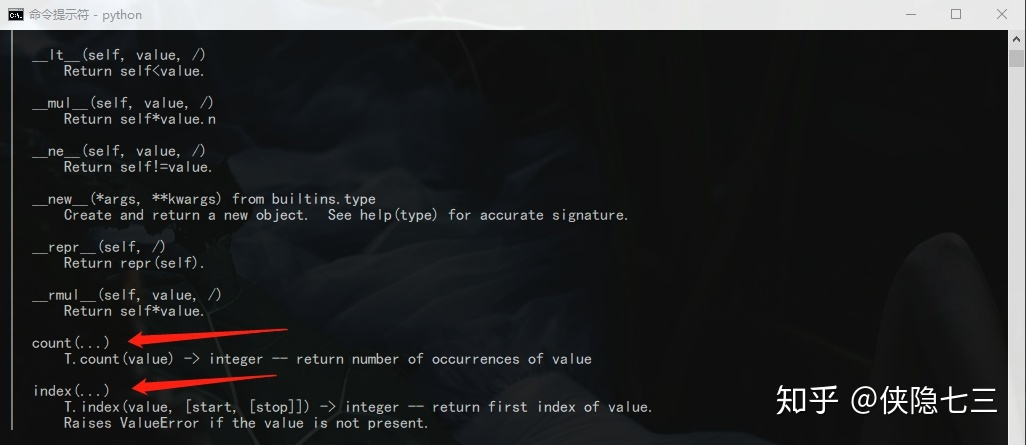# python最佳入门教程(22): 元组操作方法

## 2 源码实例

GiantFurnace/python-basic-tutorial​github.com## 3 元组操作方法

tuple_variable = (1,2,'python')
element = tuple_variable # element的值为1

numbers = (1,2,3)
numbers = numbers[0:2] # numbers的值为(1,2)
# 此时numbers指向的是切片后的新元组

nubers = (1,2,3)
names = ("bruce", "green")
result = numbers + names # result的值为(1,2,3,"bruce", "green")

nubers = (1,2,3)
numbers = numbers * 3 # numbers的值为(1,2,3,1,2,3,1,2,3)

numbers = [1,2,3]
numbers = 2  # 合法操作，对列表按索引进行修改
numbers = (1,2,3)
numbers = 2 # 非法操作，系统抛出异常numbers = (1,2,3,3,2,1)
numbers.index(1) # 输出为0
numbers.index(1,1) # 输出为5,从索引位置1处开始查找元素1的索引

numbers.count(3) # 统计元素3的个数，输出为2

## 4 本节知识要点

(1) 元组一种顺序存储的序列结构
(2) 列表是动态的，元组是静态的
(3) 不能对元组进行添加，修改，删除​​​​​​​

©️2019 CSDN 皮肤主题: 技术黑板 设计师: CSDN官方博客